# How much do you need to live a month in a month?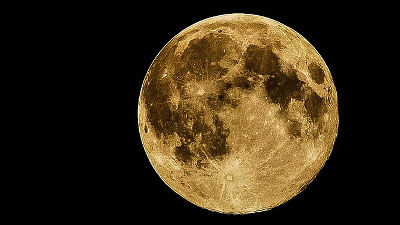SpaceX's Eulon Mask is ongoing plan to migrate humanity to MarsHowever, if you calculate roughly how much it cost when you get into the "moon" of a familiar celestial body that is nearest without saying Mars, when you live for a year, it will look something like this.

How Much Would it Cost to Live on the Moon? - YouTube

Going to the moon with a rocket and even living on the moon is technically feasible enough, only the "cost" is the problem.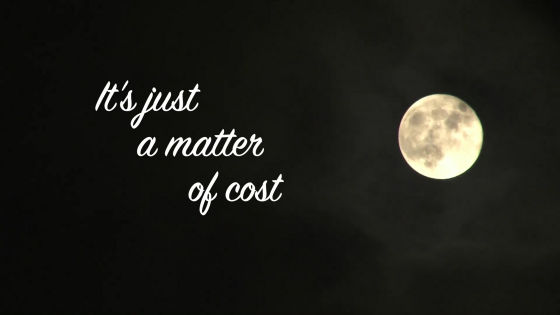The distance to the moon is about 377,000 kilometers.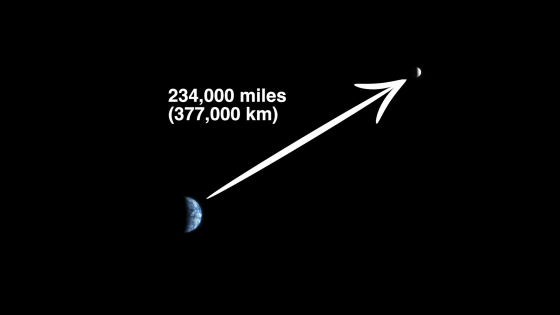I will go to this distant moon and think about how much it will cost to live a human being.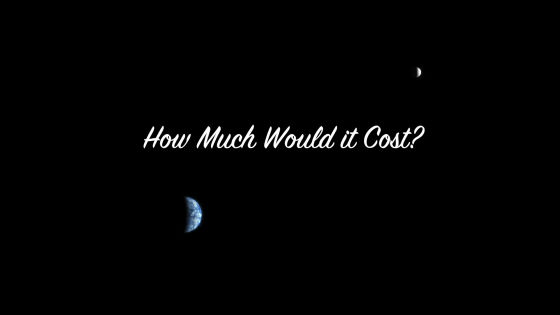The mission to examine the cost is that "four astronauts stay NASA technology for one year".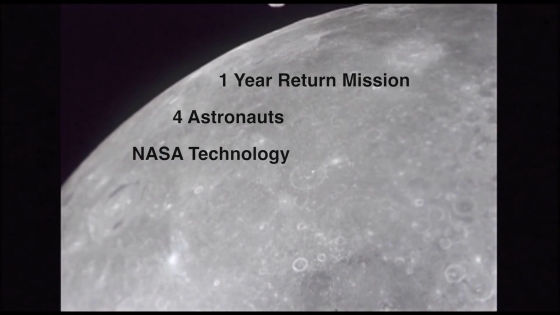First we need a rocket to be a means of transportation. Among numerous rockets, only "Saturn V" is a powerful one that can carry humans to the moon. Unfortunately, however, all 15 Saturn Vs are retired.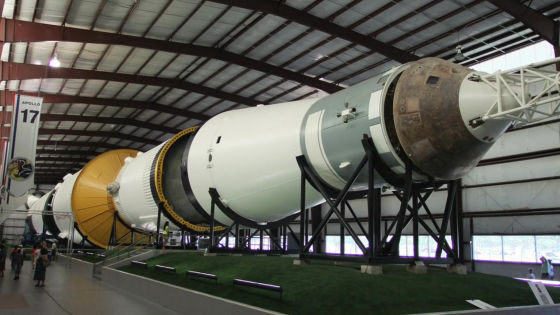The loading capacity of Saturn V was about 40,000 kilograms, and the launch cost was 189 million dollars (about 60 billion yen) at the time of 1971.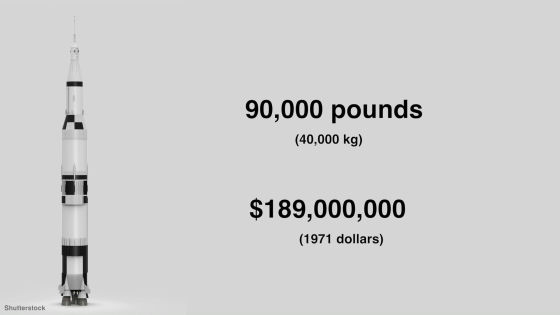This launch cost jumps to \$ 1,123 million (about 110 billion yen) in 2016.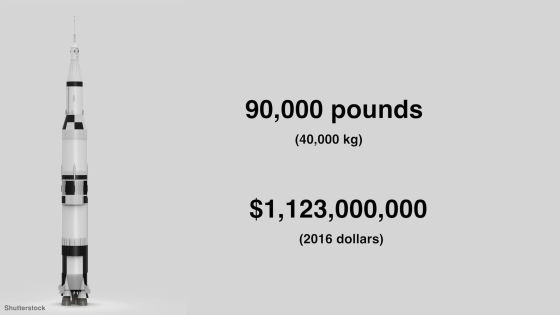The cost of carrying heavy items that can be calculated from here is up to 5700 dollars per kilogram (about 580,000 yen).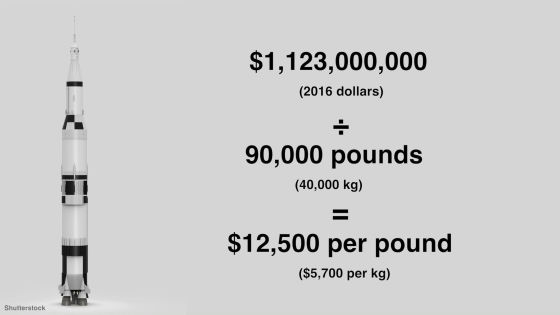Assuming that the average astronaut weight is 72 kilograms, the cost of carrying people to the moon can be calculated as 2 million dollars (about 200 million yen). But there is a problem with this calculation.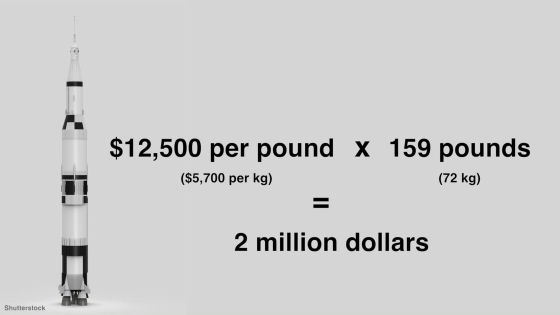Because it does not include R & D expenses that produce Saturn V.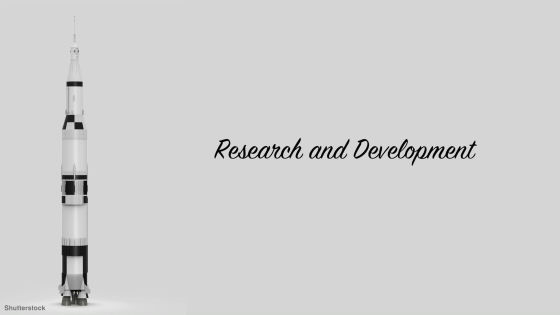In light of the monetary value of 2016, the launch cost of Saturn V was 3.8 billion dollars (about 380 billion yen)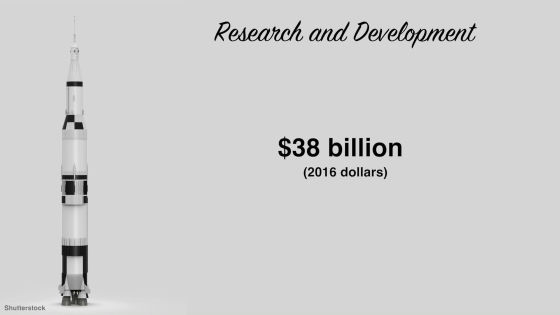Since we launched 13 times in total, it costs a total of 4 billion dollars (about 400 billion yen).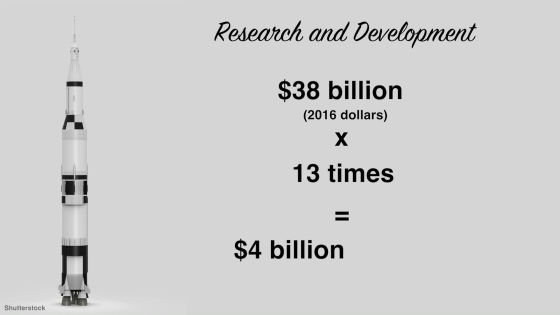So, the launch cost per kilogram can be calculated as \$ 97,000 (about 97,000 yen).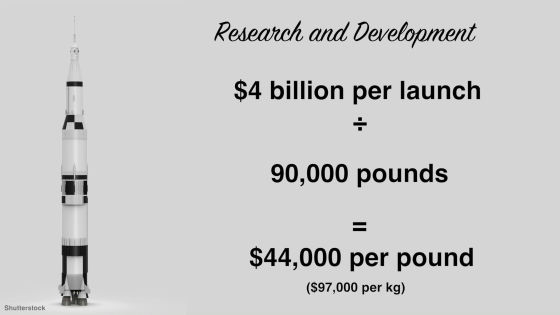Of course you can not just arrive at the moon if you think to live in the moon. I need to land on the moon.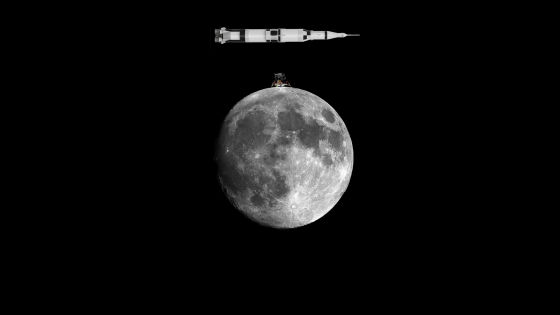In the Apollo project, we have made 12 units "Lunar Lander" for landing on the moon, which cost 20 billion dollars (about 1.8 trillion yen) in terms of 2016. In other words, it costs 1.5 billion dollars (about 150 billion yen) per Lunar Lander.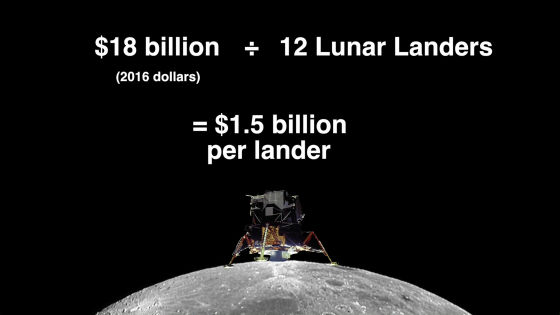For the landing of four astronauts, two Lunar Landers are needed.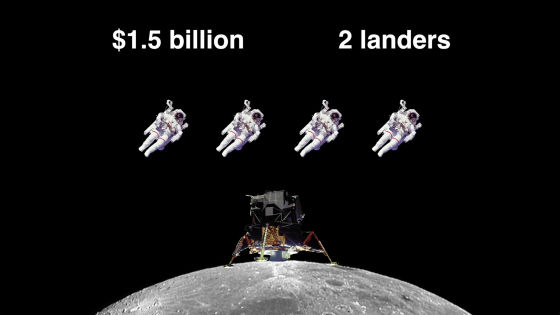So, including the cost of launching two Saturn V ... ...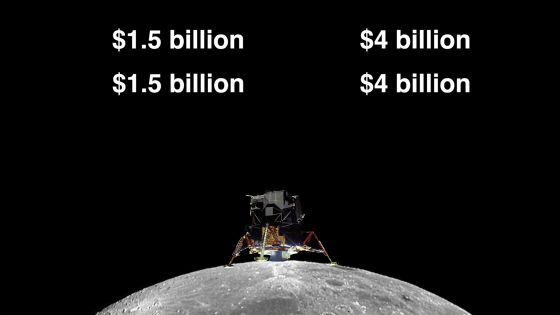The cost for landing in the month is 11 billion dollars (about 1.1 trillion yen).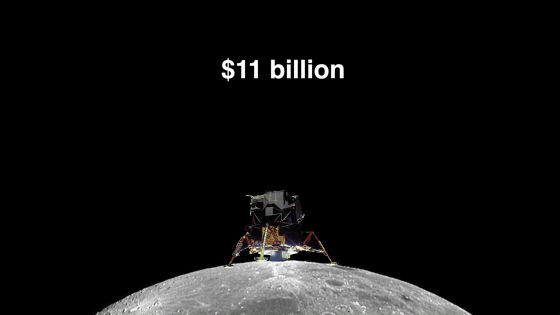During the Apollo project period, NASA was developing an unmanned landing system called "LM Truck".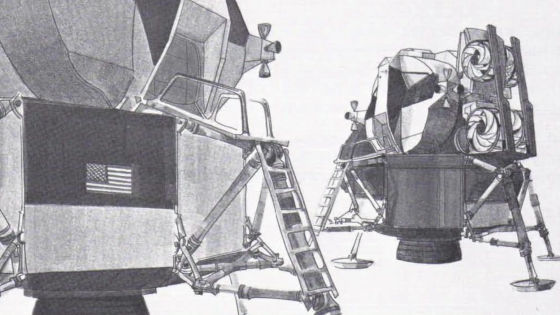We carry 2 LM Trucks of 5000 kg per machine.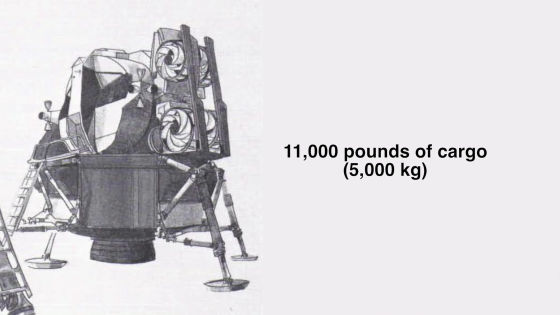The cost to carry it to the moon with LM Truck will be \$ 144,000 per kilogram (about 14 million yen).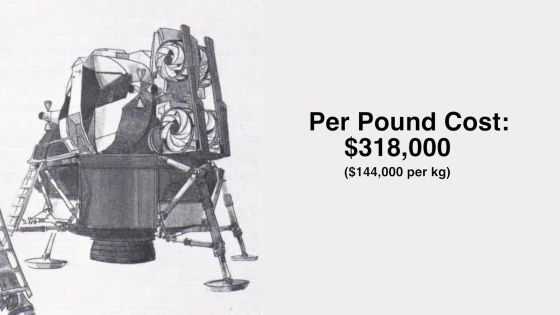Living costs are obviously required for astronauts arriving on the moon to live. For this calculationCSISHas published detailed simulation results.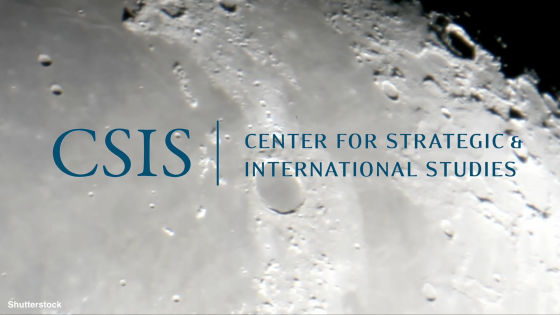According to it, the construction cost of the facilities where the four astronauts live is 20 billion dollars (about 2 trillion yen) and the launch cost is 4 billion dollars (about 400 billion yen), so the total is about 24 billion dollars (about 2 To the unrealistic amount of 400 trillion yen).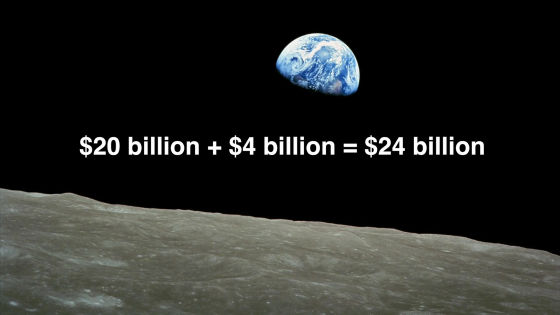Instead of constructing facilities there,Bigelow AirspaceOf the spacecraft "B330" for living space.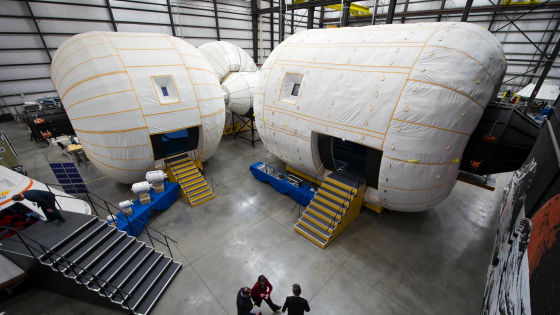Within B330 you can send a comfortable space life like this.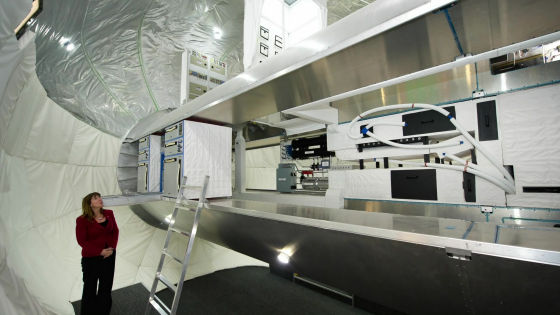Since the rental cost of B330 is \$ 1.25 million (125 million yen) per day, the cost per year will be \$ 465 million (about 47 billion yen)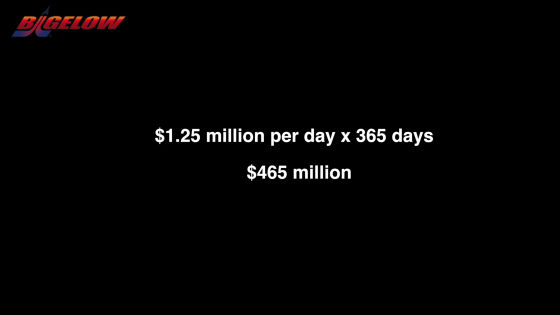The weight of B330 is about 20,000 kilograms, and for transportation it requires 4 LM Trucks and 2 Saturn V ......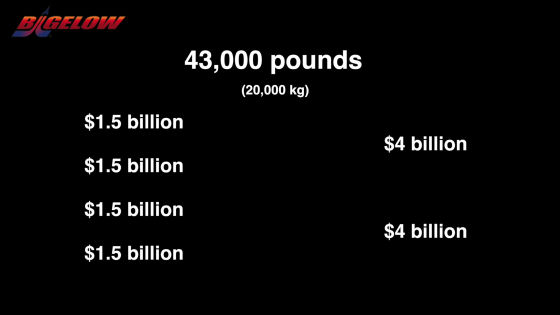A total of 14 billion dollars (about 1.4 trillion yen) is required.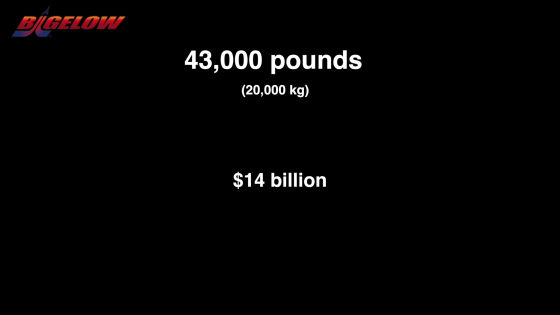Food is indispensable for living in the month.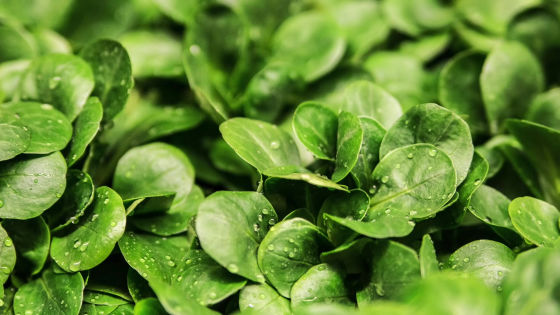The best way to obtain food is to grow plants at the moon.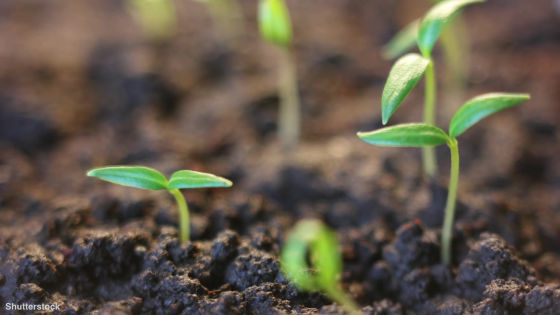The plant plant system studied by the University of Arizona can produce 5.5 pounds (about 2.5 kilograms) of plants per day.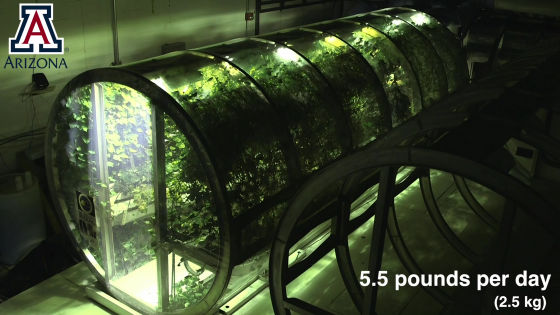Given nutrition and balance, if we decided to make 4.3 pounds of potatoes (about 2 kg), 0.8 pounds of tomatoes (about 360 grams) and 0.4 pounds of strawberries (about 180 grams) ......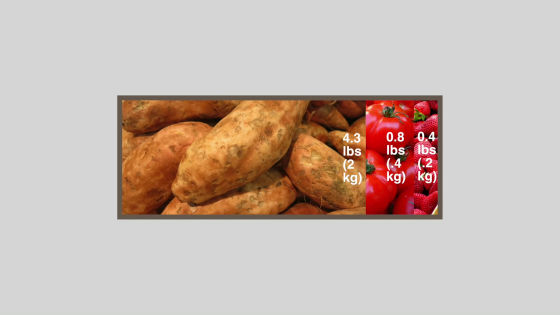A total of 1,800 kcal can be produced on a single day.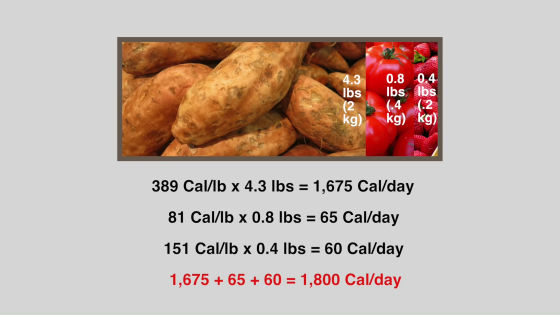Because four astronauts need a total of 12,000 kcal a day ....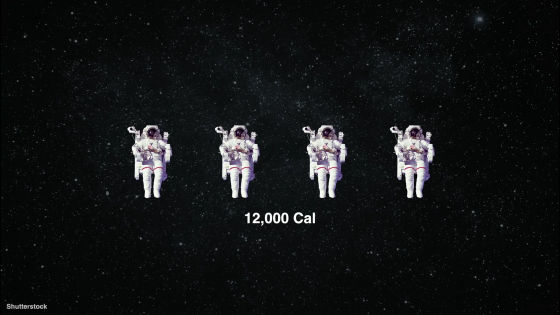Approximately 7 plant plant systems will be required.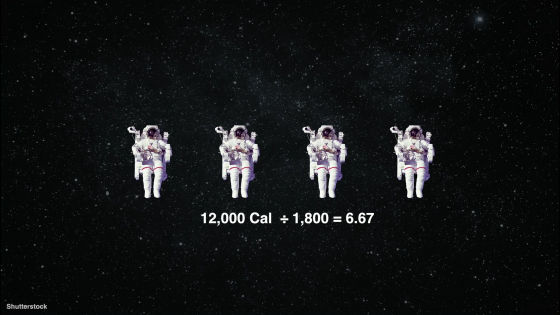Each plant plant system requires 0.2 pounds (about 90 grams) of fertilizer per day, totaling 510 pounds (about 230 kilograms) in a year.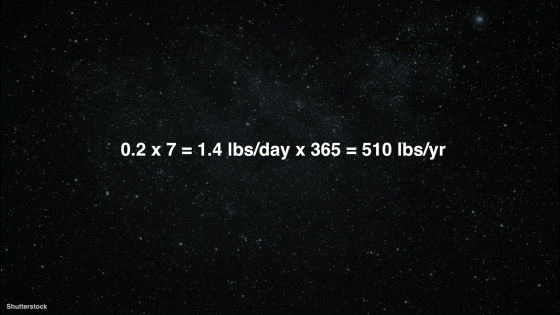Weight of the plant plant system is 2,200 pounds (about 1000 kilograms) per body and 7 bodies are needed, so 15,500 pounds (about 7000 kilograms)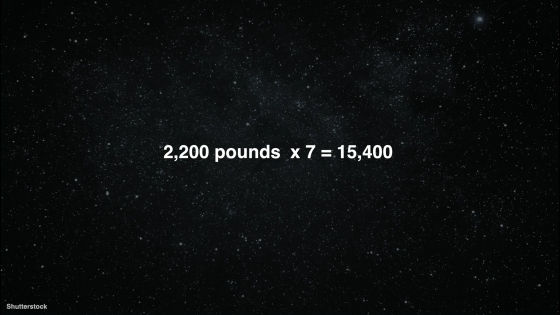This transportation cost is 4.9 billion dollars (about 490 billion yen), and further fertilizer, 162 million dollars (about 16 billion yen) will be added.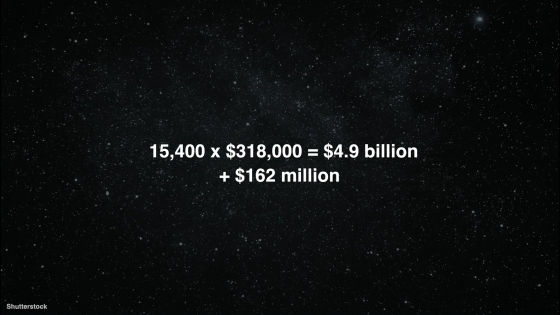How to use water in ISS is a great reference for living in the month.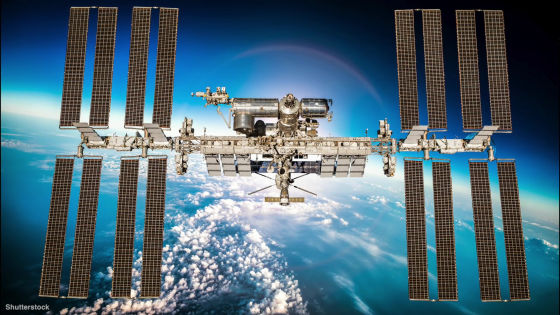With reference to ISS, the astronaut needs 18,000 kilograms of water per month in a month.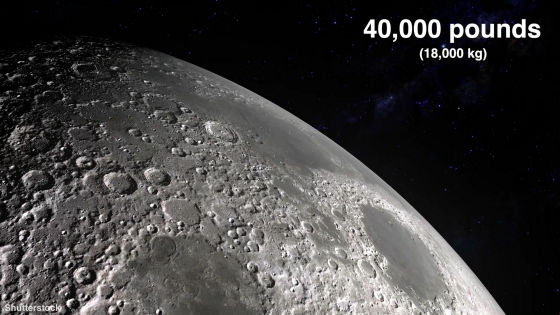This can be reduced to 2,300 kilograms by using the water circulation recycling system.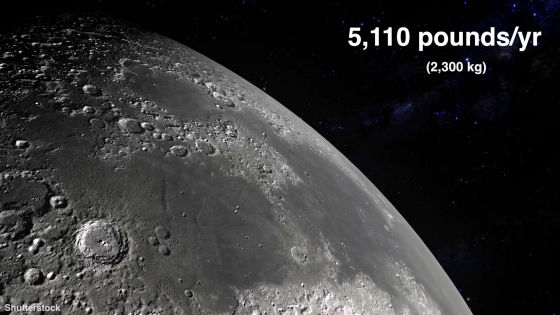However, the amount of water used in the plant plant system is 3100 kilograms.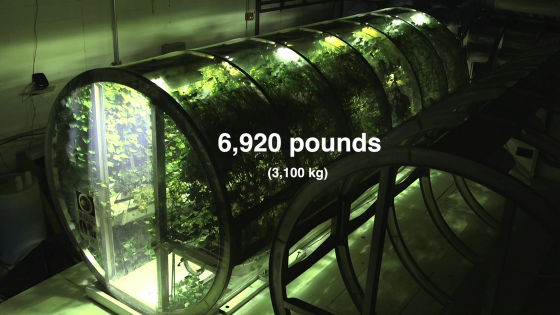So the amount of water required per month in a year is 5400 kilograms.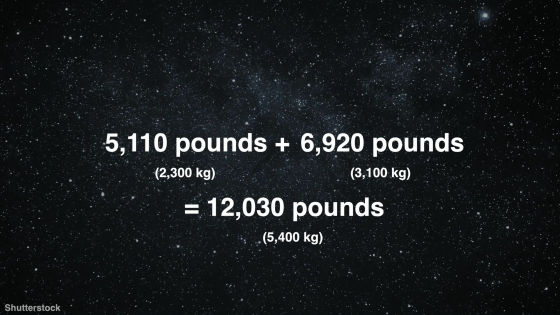Water transportation cost will be 3,825 million dollars (about 390 billion yen)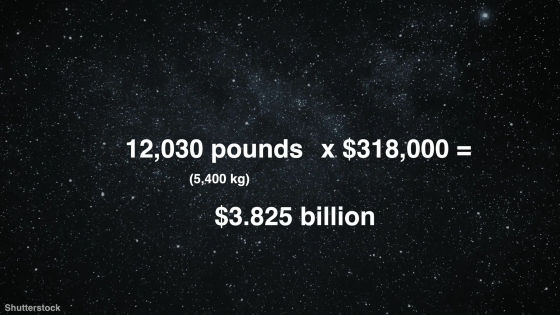Also, you need to prepare the air so that the astronauts can breathe in the moon.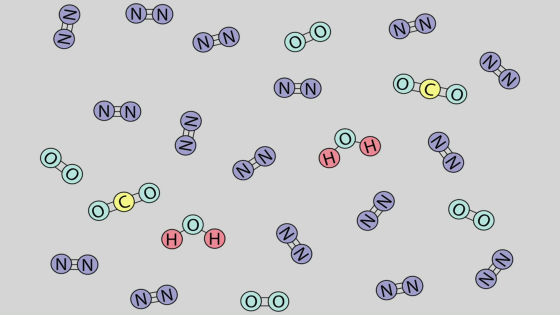Since the amount of oxygen required for adults is 1.85 pounds (about 840 grams) per day, the cost of transporting oxygen for four people in a year is \$ 860 million (about 86 billion yen).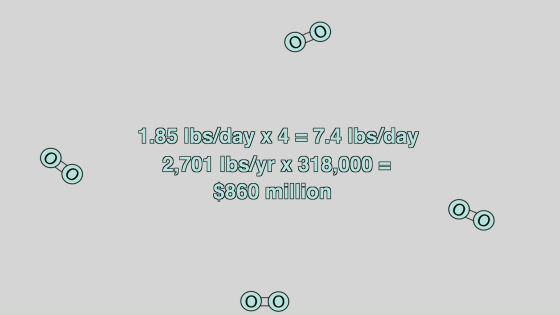But fortunately the plant plant system produces oxygen.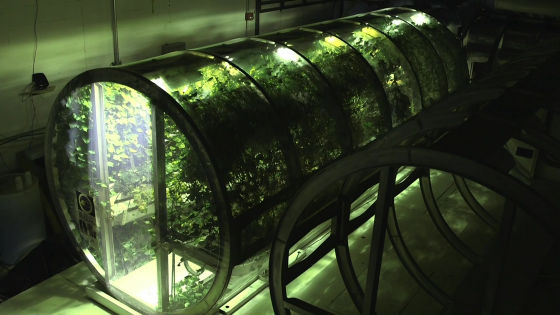The plant plant system produces 5.25 pounds (about 2.4 kilograms) of oxygen per day,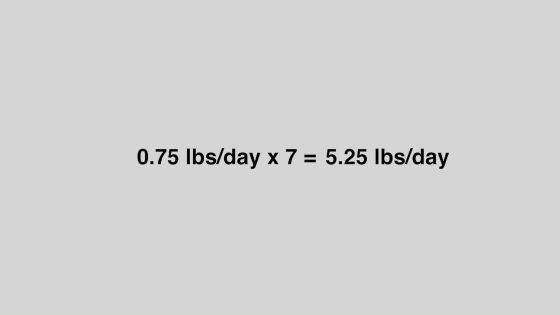Therefore, the amount of oxygen required for the daily less than 2.15 pounds (about 980 grams)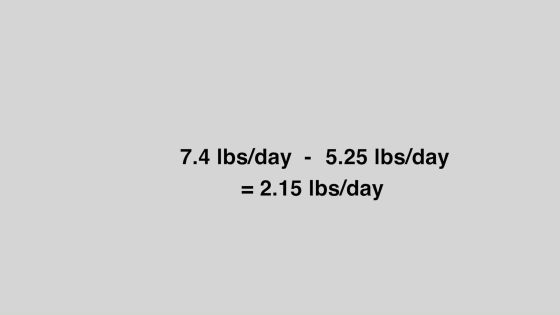Since the oxygen required for one year is 785 pounds (about 360 kilograms), the transportation cost has been reduced to 250 million dollars (about 25 billion yen).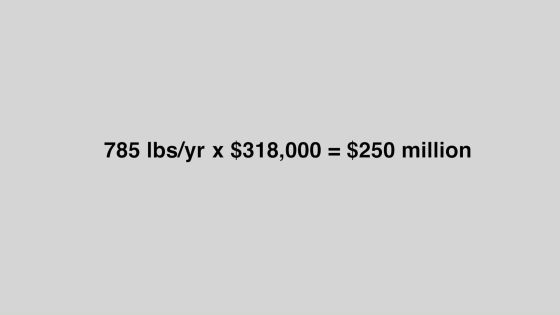Next you need energy.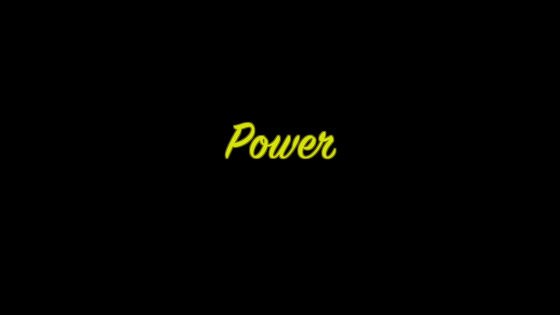The best way to procure energy in the month is nuclear power generation.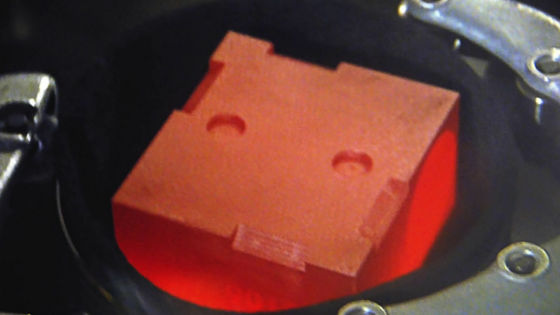For small nuclear power plants that can be used in the month, NASA is only proposing and it is still undeveloped but estimates the cost to be 1.4 billion dollars (about 140 billion yen).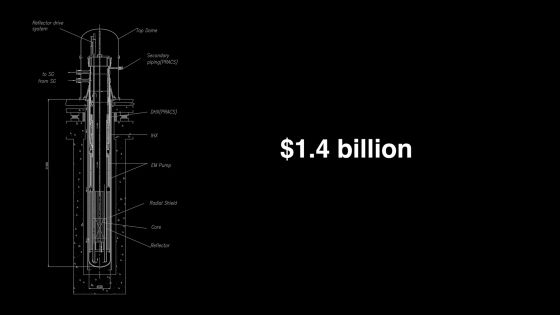All the necessary expenses have been completed. Before announcing the total amount, I will mention not the NASA but the private space development enterprises.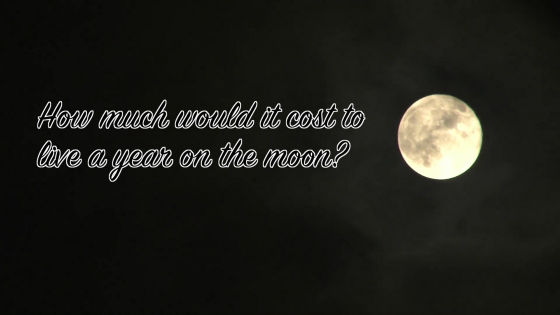SpaceX led by Eylon Mask ......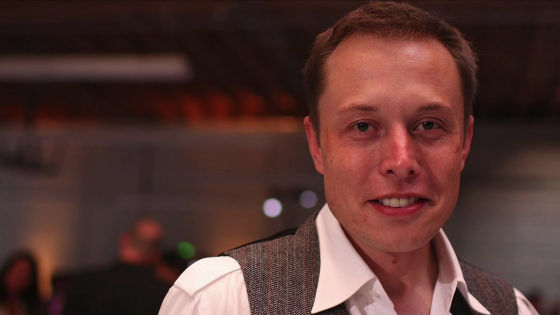I am about to send a 100,000 kilogram rocket to Mars.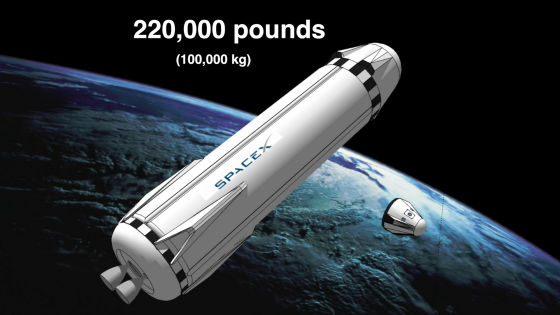Its ultimate goal is to emigrate to Mars. As private space developers such as SpaceX appear one after another, the cost of traveling to space will dramatically decrease in the future.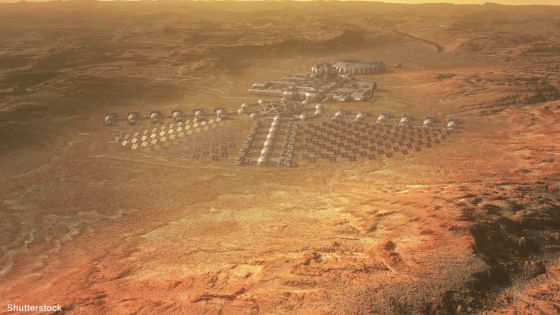The total sum of the costs necessary for four astronauts to move to the moon for one year at the present time by NASA technology ......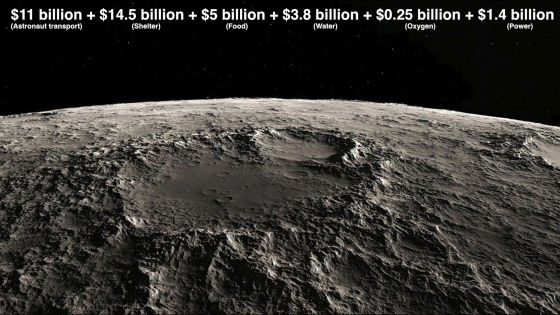Roughly estimate \$ 36 billion (about 3.6 trillion yen)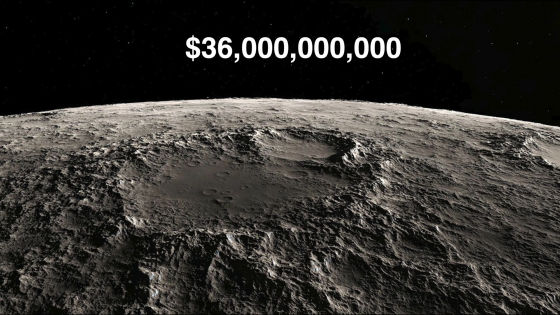It got a surprising amount of money that you need \$ 1138 (about 110,000 yen) if you fix it per second.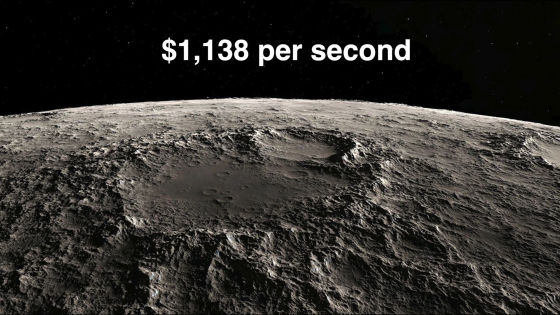in Note,   Science,   Video, Posted by darkhorse_log